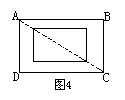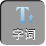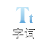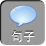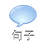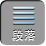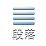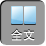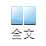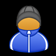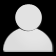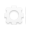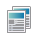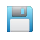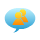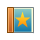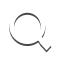-AA+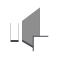1）首先确定最大边（如：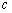）；

2）计算出最长边的平方和另两边（如：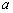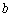）的平方和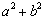3）比较最长边的平方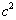和另两边的平方和，如果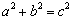，则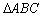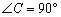的直角三角形，如果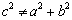，则不是直角三角形

1.如图 1，在四边形 ABCD中， AB: BC: CD: DA= 2:2:3:1，且∠ ABC= 90°，求∠ DAB的度数.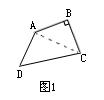AB: BC: CD: DA= 2:2:3:1= k，则 AB= 2 kBC= 2 kCD= 3 kDA= k.

RtABC中，∵ AB= BC= 2 k，∴△ ABC为等腰三角形.

∴∠ BAC= 45°，且 AC 2= AB 2BC 2= 8 k 2.

∴∠ DAC= 90°.

∴∠ DAB=∠ DAC＋∠ BAC= 90°45°= 135°.

2.如图 2，在四边形 ABCD中，已知∠ B= 90°AB= 3BC= 4CD= 12AD= 13，求四边形 ABCD的面积.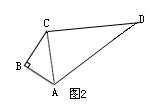RtABC中， AC 2= AB 2BC 2= 3 24 2= 25.

AC 2CD 2= 25144= 169= AD 2

∴△ ACD为直角三角形.

S四边形 ABCD= SABCSACD=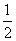× AB× BC× CD× AC=× 3× 4× 5× 12= 36.

3.如图 3，在△ ABC中， AB= 17 cmBC= 16 cmBC边上的中线 AD= 15 cm.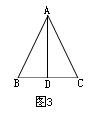AD 2BD 2= 15 28 2= 289= AB 2

RtADC中，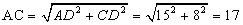(cm).

AB= AC.

4.如图 4，这是一个农民建房时挖的地基平面图，按标准应为矩形，他在挖完后测量了一下，发现 AB= CD= 8 mAD= BC= 6 mAC= 9 m，请你帮他检查一下挖的长方形是否合格.